# Information, source of

(diff) ← Older revision | Latest revision (diff) | Newer revision → (diff)

An object producing information that could be transmitted over a communication channel. The information produced by a source of informationis a random variabledefined on some probability space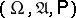, taking values in some measurable space, and having a probability distribution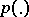.

Usuallywhere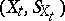are copies of one and the same measurable space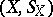and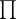is the direct product ofasruns through a setthat is, a rule, either a certain interval (finite, infinite to one side, or infinite to both sides) on the real axis, or some discrete subset of this axis (usually,or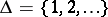). In the first of these cases one speaks of a continuous-time source of information, while in the second — of a discrete-time source of information. In other cases, a random variable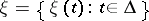with values inrepresents the information. In applicationsis treated as the information produced by the source at the moment of time. The samples of random variables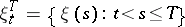are called the segments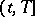of information.

Sources of information can be divided into various classes, depending on the type of information, i.e. of the random processproduced by the source. E.g., ifis a random process with independent identically-distributed values, or if it is a stationary, an ergodic, a Markov, a Gaussian, etc., process, then the source is called a source of information without memory, or a stationary, ergodic, Markov, Gaussian, etc., source.

One of the problems in the theory of information transmission (cf. Information, transmission of) is the problem of encoding a source of information. One distinguishes between, e.g. encoding of a source by codes of fixed length, by codes of variable length, encoding under given accuracy conditions, etc. (in applications some encoding problems are called quantization of information, contraction of information, etc.). E.g., letbe a discrete-time source of information without memory producing information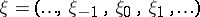with componentsthat take values in some finite set (alphabet). Suppose there is another finite set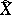(the set of values of the componentsof the information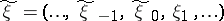reproduced). An encoding of volumeof a segment of information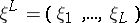of lengthis a mapping of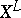into a set ofelements of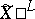. Let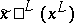be the image ofunder such a mapping (is the direct product ofcopies of). Suppose further that the exactness of reproducibility of information (cf. Information, exactness of reproducibility of) is given by a non-negative real-valued function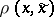,,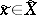, a measure of distortion, for which its average measure of distortion of encoding is given by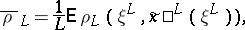(1)

where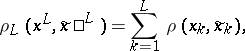if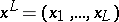and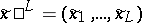. The quantity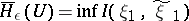(2)

is the-entropy of a source of information without memory. Here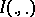is the amount of information (cf. Information, amount of), and the infimum is over all compatible distributions of pairs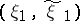,,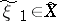, for which the distribution of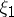coincides with the distributions of the individual components ofand for whichThe encoding theorem for a source of information. Letbe the-entropy of a discrete sourcewithout memory and with a finite measure of distortion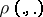; let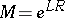. Then: 1) for any,,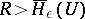, and all sufficiently large, there is an encoding of volumeof the segments of lengthsuch that the average distortion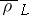satisfies the inequality; 2) if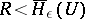, then for any encoding of volumeof segments of lengththe average distortionsatisfies the inequality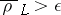. This encoding theorem can be generalized to a wide class of sources of information, e.g. to sources for which the spaceof values of the components is continuous. Instead of an encoding of volumeone speaks in this case of quantization of volume of the source of information. It must be noted that the-entropy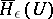entering in the formulation of the theorem coincides, for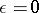and measure of distortion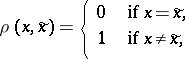as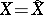, with the rate of generation of information by the given source (cf. Information, rate of generation of).

How to Cite This Entry:
Information, source of. Encyclopedia of Mathematics. URL: http://encyclopediaofmath.org/index.php?title=Information,_source_of&oldid=47352
This article was adapted from an original article by R.L. DobrushinV.V. Prelov (originator), which appeared in Encyclopedia of Mathematics - ISBN 1402006098. See original article